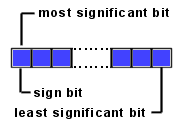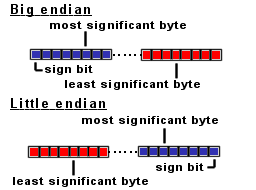# Java Quick Guide

This guide contains useful Java information.

## Java integer type.

Information
Integer types are so called signed two-complement numbers. The 2 complement scheme allows both positive and negative integers to be represented. The most significant bit of a two complement number is its sign bit. The sign bit is 1 for negative numbers and 0 for positive numbers and for 0.In Java the bytes are big-endian ordered, which means the most significant BYTE (where the sign bit is located) is always on the left side:Integer values are stored in 4 bytes and contains positive or negative integer numbers.

The integer value range is: 232 = 4294967296

Half of this number (4294967296) are positive numbers ranging from: >=0 and <=2147483647
For example:
0x0000 0x0000 = 0
0x0001 0x0001 = 1
0x7FFF 0xFFFF = 2147483647

The other half are negative numbers ranging from: >=-2147483648 and <0
For example:
0xFFFF 0xFFFF = -1
0x8000 0x0000 = -2147483648

To convert negative decimal values into hexadecimal values:
value = 232 + (neg_dec_value)
value = 4294967296 + (neg_dec_value)

For example:
neg_dec_value= -1
value = 4294967296 + (neg_dec_value) = 4294967296 + (-1) = 4294967295
The new decimal value 4294967295 equals 0xFFFF 0xFFFF (Use your Windows calculator, to verify this).

 Type Min value Max value Integer -2147483648 2147483647